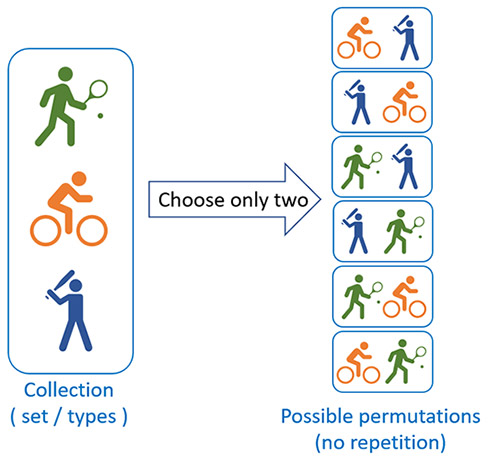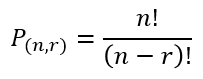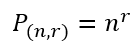# Permutations Calculator

Use this calculator to easily calculate the number of permutations given a set of objects (types) and the number you need to draw from the set.

### Calculation results

125 Permutations
Share calculator:

Embed this tool:
get code

## What is a permutation?

A permutation is a way to select a part of a collection, or a set of things in which the order matters and it is exactly these cases in which our permutation calculator can help you. For example, if you have just been invited to the Oscars and you have only 2 tickets for friends and family to bring with you, and you have 10 people to choose from, and it matters who is to your left and who is to your right, then there are exactly 90 possible solutions to choose from. Permutations come a lot when you have a finite selection from a large set and when you need to arrange things in particular order, for example arranging books, trophies, etc.

Calculating permutations is necessary in telecommunication and computer networks, security, statistical analysis. Systems like phone numbers, IP addresses, IBANs, etc. are designed based on the knowledge of the maximum available permutations versus the expected use. A given phone area prefix can only fit in that many numbers, the IPv4 space can only accommodate that many network nodes with unique public IPs, and an IBAN system can only accommodate that many unique bank accounts.

Here is a more visual example of how permutations work. Say you have to choose two out of three activities: cycling, baseball and tennis, and you need to also decide on the order in which you will perform them. The possible permutations would look like so:Permutation calculations are important in statistics, decision-making algorithms, and others.

## How to calculate permutations?

To calculate the number of possible permutations of r non-repeating elements from a set of n types of elements, the formula is:The above equation can be said to express the number of ways for picking r unique ordered outcomes from n possibilities. If the elements can repeat in the permutation, the formula is:In both formulas "!" denotes the factorial operation: multiplying the sequence of integers from 1 up to that number. For example, a factorial of 4 is 4! = 4 x 3 x 2 x 1 = 24.

## Permutation with repetition

In some cases, repetition of the same element is allowed in the permutation. For example, locks allow you to pick the same number for more than one position, e.g. you can have a lock that opens with 1221. Or you can have a PIN code that has the same number in more than one position. The formula for calculating the number of possible permutations is provided above, but in general it is more convenient to just flip the "with repetition" checkbox in our permutation calculator and let it do the heavy lifting.

Another common example concerns dice probabilities. If two six-sided dice are thrown together, one needs to estimate the number of possible ways they may come up. This a case of randomly drawing two numbers out of a set of six, and since the two may end up being the same (e.g. double sixes) it is a calculation of permutation with repetition. The answer in this case is simply 6 to the power of 2, 6 · 6 = 36 possible permutations of the two dice rolls.

## Permutations vs combinations

The difference between combinations and permutations is that permutations have stricter requirements - the order of the elements matters, thus for the same number of things to be selected from a set, the number of possible permutations is always greater than or equal to the number of possible ways to combine them. With combinations we do not care about the order of the things resulting in fewer combinations. Permutations are for ordered lists, while combinations are for unordered groups. For example, if you are thinking of the number of combinations that open a safe or a briefcase, then these are in fact permutations, since changing the order of the numbers or letters would result in an invalid code.

Very often permutations are mistaken for combinations, at least in common language use. For example, a "combination lock" is in fact a "permutation lock" as the order in which you enter or arrange the secret matters. In computer security, if you want to estimate how strong a password is based on the computing power required to brute force it, you calculate the number of permutations, not the number of combinations.

#### Cite this calculator & page

If you'd like to cite this online calculator resource and information as provided on the page, you can use the following citation:
Georgiev G.Z., "Permutation Calculator", [online] Available at: https://www.gigacalculator.com/calculators/permutation-calculator.php URL [Accessed Date: 01 Apr, 2023].